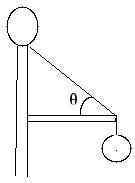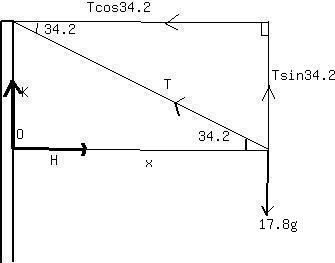# A 17.8 kg flood light in a park is supported at the end of a horizontal beam of negligible mass that is hinged to a pole. A cable connected to the beam helps to support the lightpostillan4 2021-02-04 Answered

A 17.8 kg flood light in a park is supported at the end of a horizontal beam of negligible mass that is hinged to a pole. A cable connected to the beam helps to support the light. (In thefigure, $\theta$ = 34.2 degrees. Find (A) The tension in the cable and (B) the horizontal and (C) vertical forces exerted on the beamby the pole.(A) 320 N
(B) 250 N
(C) 0

You can still ask an expert for help

• Questions are typically answered in as fast as 30 minutes

Solve your problem for the price of one coffee

• Math expert for every subject
• Pay only if we can solve iti1ziZWeight $=mg$
Take $g=9.8N/kg$
Weight of the floodlight $=17.8\cdot 9.8=174.44$ N
Let the length of the beam be x.
Perpendicular distance from the force T to the the point O
$Moment=force\cdot$ perpendicular distance from the line ofaction of the force to the pivot
Anti clockwise moment = clockwise moment

resolving horizontal forces,